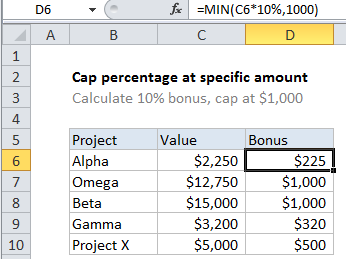## Excel Office

Excel How Tos, Tutorials, Tips & Tricks, Shortcuts

# Calculate cap percentage at specific amount in Excel

This tutorial shows how to calculate a percentage based value capped at a specific amount using MIN function. See illustration below:

## Formula

`=MIN(A1*percent,1000)`## Explanation

In the example shown, the formula in D6 is:

`=MIN(C6*10%,1000)`

### How this formula works

This formula takes advantage of the fact that the MIN function works fine with small sets of data, even two values.

Also See:   Maximum value in Excel

Inside MIN, the value in C6 is multiplied by 10%, and the result appears at the first number given to MIN. The number 1000 is supplied as the second value.

The MIN function then returns the smaller of the two values.